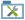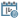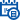Create a Complex Date Calculation

To create a complex date calculation, do the procedure in this topic.

How to Start

1. , do one of these:
2. Do one of these:
• Add an activity:
1. In the Process Builder, in the Activity Library, open the Utilitiestab.
2. On the Utilitiestab, drag the Calculate Date Differenceactivity onto your process.
• Change an activity:
1. In your process, double-click your activity.
3. Click Calculate Date Difference.

Procedure

1. On the Calculate Date Difference screen on the Input tab, complete this field as necessary.
Field Name Value

Input Date Time

Function:
Specifies the reference date for your date calculation.

Two Date Time fields are available: Input Date Time 1 and Input Date Time 2.

Accepted Values:
• Custom Attributes - Specifies a process data variable that stores the date-time value and your date-time format.

You can use Process Data screen to specify a process data variable.

You can also use a custom date-time format. For more information, refer to Customize Your Date-Time Format.

• Static - Specifies a static date and time.
• Now - Specifies the date and time your activity starts.
Default Value:
Custom Attribute
2. Click the Operation tab.
3. On the Operation tab, select Calculate Difference, and complete these fields as necessary.
Field Name Value

Compare Difference

Function:
Specifies how to compare the difference between the two Input Date Time values.
Accepted Values:
1. The operator used to compare the two Input Date Time values:
• <= - Less than or equal to.
• >= - Greater than or equal to.
• = - Equals
• <> - Not equal to.
2. An integer, or a process data variable that stores an integer.

You can use Process Data screen to specify a process data variable.

3. The time unit.

Options are:

• Seconds
• Minutes
• Hours
• Days
• Weeks
• Months
• Years
Default Value:
None
Accepts Process Data Variables:
Yes

Calculation Type

Function:
Specifies how the difference between the Input Date Time 1 and Input Date Time 2 is calculated.
Accepted Values:
• Real - The actual difference between the two DateTime values. The result depends upon the option set in the Result field.
• Relative - The relative difference between the two DateTime values. This setting is useful for situations when an action is allowed, for example, once per week or once per month.
Default Value:
Real
Example:
The difference between the dates Sat 29-Dec-2007 23:50:00 and Wed 02-Jan-2008 00:00:00 is calculated as follows. Note that these dates are 4 days apart, but occur in different years, different months, and different weeks.
• When you select the Real option the difference is calculated based on the actual number of days (and hours and minutes) between the two dates: 4 days and 10 minutes.
• When you select the Relative option, the difference is 4 days, 1 week or 1 month, or 1 year, depending upon the time unit specified.
4. Click the Output tab.
5. On the Output tab, complete these fields as necessary.
Field Name Value

Result Boolean

Function:
Specifies the name of the variable that stores the Boolean result returned from the Compare Difference date calculations.

If the Compare Difference option is enabled, the difference is evaluated as a Boolean result, and the Boolean value is associated with the variable that appears in this field.

Accepted Values:
that stores a Boolean Date Time result.
Default Value:
None
Accepts Process Data Variables:
Yes

Date String

Function:
Specifies the process data variable that stores the result received from the date calculations if it is in the Date Time format.

The results of the date calculations that changes from the Date Time format to a String. The format of the string is specified in the list.

Accepted Values:
that stores the result in the selected String format.
Default Value:
None
Accepts Process Data Variables:
Yes

Difference

Function:
Specifies the result returned from the Compare Difference date calculations. In terms of a number of time units, such as 5 seconds, or 10 days.

If Compare Difference is enabled, the difference is stored as a variable with the name that appears in this field. The value is the output value of the comparison.

Accepted Values:
• that stores the value of the comparison result.
• The time unit.

Options are:

• Seconds
• Minutes
• Hours
• Days
• Weeks
• Months
• Years
Default Value:
• None
• Days
Accepts Process Data Variables:
Yes

Result

Function:
Specifies the type of value to return for the date calculations where the Calculation Type is set as Real.
Accepted Values:
• Decimal - Returns the difference as is, including any decimals.
• Whole Number - Returns the integer part of the result by removing the decimal part.
• Rounded - Rounds up (>=0.5) or rounds down (<0.5) the result.
Default Value:
None